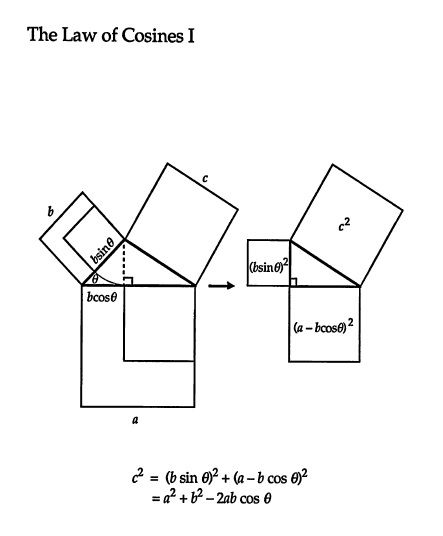# Partial Derivatives of the cosine rule.

#### Kris

##### New member
Partial Derivatives

Hi all I was wondering if anyone could help me with this problem. I have a triangle that has a = 13.5m, b = 24.6m c, and theta = 105.6 degrees.

Can someone remind me of what the cosine rule is?

Also (my question is here)

From the cosine rule i need to find:

• the partial derivative of c with respect to a?
• the partial derivative of c with respect to b?
• the partial derivative of c with respect to theta?

How would i go about finding these partial derivatives for these? Do I need to know what the cosine rule is and what the value of c is?

How to find the partial derivatives?

Any help will be appreciated.

Last edited by a moderator:

#### Klaas van Aarsen

##### MHB Seeker
Staff member
Hi all I was wondering if anyone could help me with this problem. I have a triangle that has a = 13.5m, b = 24.6m c, and theta = 105.6 degrees.

Can someone remind me of what the cosine rule is?

Also (my question is here)
From the cosine rule i need to find

• the partial derivative of c with respect to a?
the partial derivative of c with respect to b?
the partial derivative of c with respect to theta?

How would i go about finding these partial derivatives for these? Do I need to know what the cosine rule is and what the value of c is?

Any help will be appreciated
Welcome to MHB, Kris!The cosine rule is
$$c^2=a^2+b^2-2ab\cos \theta$$
where $\theta$ is the angle between sides a and b.

In other words,
$$c=\sqrt{a^2+b^2-2ab\cos \theta}$$
You're supposed to take the partial derivatives of this expression.
To evaluate them, you don't need the value of c.

#### Kris

##### New member
Ok so you are saying I only need the values of a,b and theta if i wish to calculate the value of c right?

Otherwise I am to calculate the partial derivatives from the formula right?

Staff member
Yep.

#### Kris

##### New member
Ok, thanks for the cosine rule.

c=sqrt(a^2+b^2−2abcos(θ))

Ok, here are my partial derivatives:

c/a = sqrt(2a+ b^2 - 2bcos(θ))

c/b = sqrt(a^2+ 2b- 2acos(θ))

c/θ = sqrt(a^2+ b^2 - 2ab)

are these correct? or am I doing something wrong?

Last edited by a moderator:

#### Klaas van Aarsen

##### MHB Seeker
Staff member
You will need to apply the chain rule in all 3 cases.
Do you know what the chain rule is?

Furthermore, do you know what the derivative of $\cos \theta$ is?

#### Kris

##### New member
is the derivative of costheta -sintheta?

what do you mean i will need to apply the chain rule? is that because we are dealing with a square root? is the derivatives i provided the inside of the chain rule? I am confused as to what you are trying to say. with the chain rule comment?

#### Klaas van Aarsen

##### MHB Seeker
Staff member
is the derivative of costheta -sintheta?
Correct!

what do you mean i will need to apply the chain rule? is that because we are dealing with a square root? is the derivatives i provided the inside of the chain rule? I am confused as to what you are trying to say. with the chain rule comment?
Yes, it is because of the square root.
Do you know the derivative of a square root?

You can't just ignore the square root and differentiate what's inside.
Instead you need to take the derivative of the square root and leave its contents intact.
And then multiply everything with the derivative of the contents.

#### Kris

##### New member
so it will look something like 1/2 ( function)^-1/2 multiplied by partial derivative required then?

#### Klaas van Aarsen

##### MHB Seeker
Staff member
so it will look something like 1/2 ( function)^-1/2 multiplied by partial derivative required then?
Yes!

#### MarkFL

Staff member
Re: Partial Derivatives

Can someone remind me of what the cosine rule is?

How to find the partial derivatives?
Any help will be appreciatedMay I ask why you have changed your original post? This makes a topic harder to follow if information that is used in subsequent posts is later removed.

#### Prove It

##### Well-known member
MHB Math Helper
I always write the cosine rule as \displaystyle \displaystyle \begin{align*} c^2 = a^2 + b^2 - 2ab\cos{(C)} \end{align*}, since it is convention to label the sides with lowercase letters and the angle opposite each side with the same capital letter.

Just for fun, here's a proof of the Cosine Rule (although they use $$\displaystyle \displaystyle \theta$$).